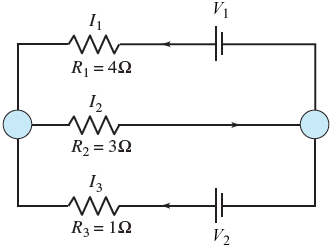## Determine the currents I1, I2, and I3 for the electrical network shown in the...

Determine the currents I1, I2, and I3 for the electrical network shown in the figure. (Assume

V1 = 3 V

and

V2 = 4 V.)

 I1 = A I2 = A I3 = ASolved